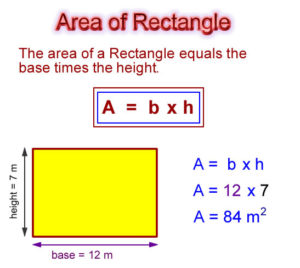# Java Program To Find Area Of Rectangle | 3 Ways

Java program to calculate the area of a rectangle. There are maybe so many methods in order to write the java program to find the area of a rectangle. Here we share the two methods, sample method -1, and sample method-2 with online compiler and execution tool.

Below are the online compiler and execution tool check it out #area of rectangle

what is a rectangle? ( Lol :-p you knew it, but just a couple of lines )

The rectangle is a plane figure with four straight sides or just four right angles.What is the formula for the area of a rectangle?

The area of a rectangle formula is :

Length X Breadth. Or Length X Width

Here goes the sample program to find or to calculate the area of a rectangle.

Output :

Here is the online execution tool!

The above program is just a simple one. since there may be several ways to represent the program. If you good at basic skills of java programming, just skip the below code explanation part and move on to the sample method-2. Where you can execute the program there by itself. Consider it has another sample method-2.

1)

– Syntax, which read the input values, in simple words, it’s an inbuilt package.

2)

– A class is a blueprint, where objects are created.

3)

– And, the program starts executing from here, the main head of the block program starts here.

4)

– scanner, which reads the input values, and system.in uses to retrieve the data from the JDK package from your system or device.

5)

– Displays, whatever you were written on that syntax, followed by second.

6)

– Double, A memory space where the user can give the values either in decimal points or without decimal points. Since we are using the ” Double “. Double can store the values up to 8 bytes, and the name os the memory is L. Consider it has same to ” B “.

7)

– The formula to find out the area of the rectangle.

8)

– Output displayed here.

We always make sure to write the java program in a simple way to the users and newbies can able to understand the program better. Lengthy codes are way better too, but those extra couple of code lines are actually not necessary.

Here is another simple method to find out the area of a rectangle, the below program is quite simple.

# Sample Method – 2 #

Note: The following program is just for a subject purpose, with your own inbuilt variables directly dumping into the program. That means a user is not required to submit the input values. Just for a subject purpose.

Output :

Actually, there is no need to use the scanner function, since there are no user inputs. The inputs values are inbuilt in a program. How simple it was right? But it is not an actual program. Just for a subject purpose.

Here, goes another sample method – 3 # Using a parse. As a matter of fact, most of the programmers or developers will not use this syntax. Just for a subject purpose, we have shared another method here. If you have any doubts related to this query do comment here, we are glad to help you out.

x

## X Star Pattern Java Program – Patterns

Java program to print X star pattern program – We have written the below print/draw ...